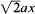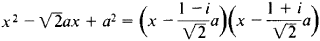# Factorization of a Polynomial

## Factorization of a Polynomial

the representation of a polynomial as a product of two or more polynomials of lower degrees. For example,

x2 – 1 = (x - 1)(x + 1)

x2 – (a + b)x + ab = (xa)(xb)

x4a4 = (xa)(x + a)(x2 + a2)

The simplest methods of factoring include the removal of a common factor—

x4 + a2x2 = x2(x2 + a2)

x (xa) – b (xa) = (xa)(xb)

the use of memorized formulas—

x2a2 = (xa)(x + a)

x3a3 = (x – a)(x2 + ax + a2)

x2 + 2ax + a2 = (x + a)2

x3 + 3ax2 + 3a2x + a3 = (x + a)3

and factoring by grouping—

x3 + ax2 + a2x + a3 = (x3 + ax2) + (a2x + a3)

= x2(x + a) + a2(x + a)

= (x + a)(a2 + x2)

x4 + a4 = (x4 + 2a2x2 + a4) – 2a2x2

= (x2 + a2)2 – ()2

= (x2 -+ a2)(x2 ++ a2)

If a polynomial of degree n

p (x) = a0 + a1x x + a2x2 + … + anxn

(an ≠ 0) has roots x1, x2, …, xn, it can be factored in the following way:

p (x) = an(xx1)…(xxn)

Here, all the factors are linear—that is, they are of the first degree. As an example, let us take the cubic polynomial x3 – 6x2 + 11 x – 6. Since it has the roots x1 = 1, x2 = 2, and x3 = 3, it can be factored as follows:

x3 + 6x2+ 11x – 6 = (x – 1)(x – 2)(x – 3)

In general, every polynomial with real coefficients can be resolved into linear and quadratic factors that also have real coefficients. An example is the factorization given above for x4+ a4. In this case, both factors are quadratic. If a is real and nonzero, the two factors can be themselves resolved only into factors with complex coefficients; for example,There exist polynomials in two or more variables of arbitrarily high degree that cannot be factored at all. An example is the polynomial xn + y, where n is any natural number. Such a polynomial is said to be irreducible.

### REFERENCE

Kurosh, A. G. Kurs vysshei algebry, 10th ed. Moscow, 1971.

A. I. MARKUSHEVICH

Site: Follow: Share:
Open / Close